Basic Mathematics

# Basic Angle Chasing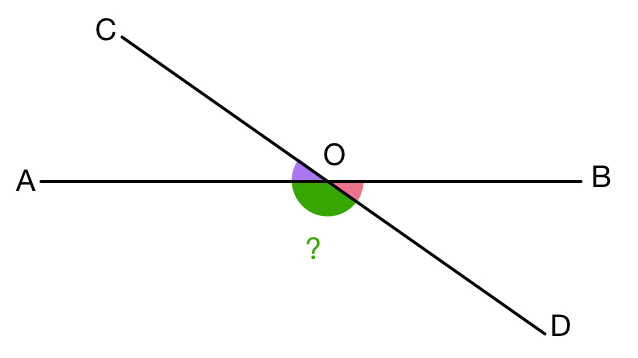Line segments $\overline{AB}$ and $\overline{CD}$ intersect at point $O.$ If $\color{#69047E}{\angle AOC}+\color{#D61F06}{\angle BOD}=78 ^\circ,$ what is the measure (in degrees) of $\color{#20A900}{\angle AOD}?$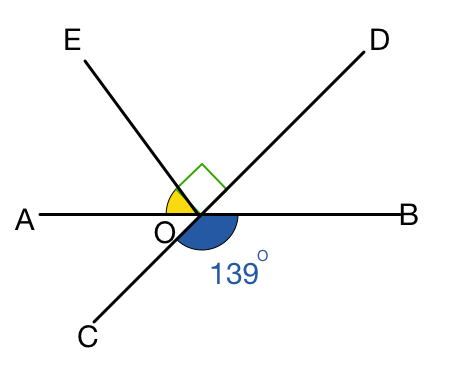Three line segments $\overline{AB},$ $\overline{CD}$ and $\overline{EO}$ intersect at point $O.$ If the blue angle $\color{#3D99F6}{\angle BOC = 139^\circ}$, what is the measure of the yellow angle?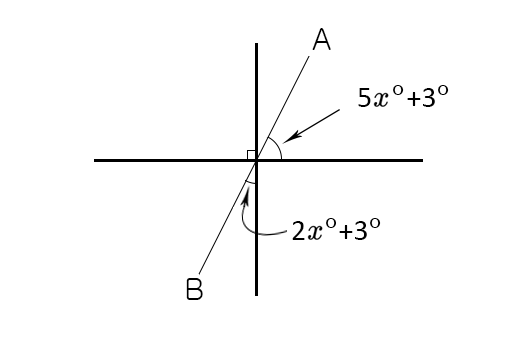Given that $\overline{AB}$ is a straight line, what is $x$?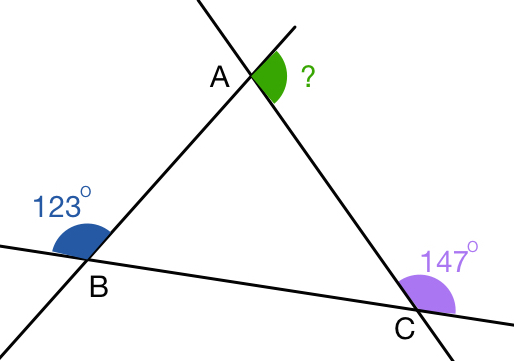In the above diagram, the purple angle at $\color{#69047E}{C}$ equals $\color{#69047E}{ 147}^\circ$ and the blue angle at $\color{#3D99F6}{B}$ equals $\color{#3D99F6} {123} ^ \circ$. What is the measure of the green angle at $\color{#20A900}{A}$?

Note: The diagram is not drawn to scale.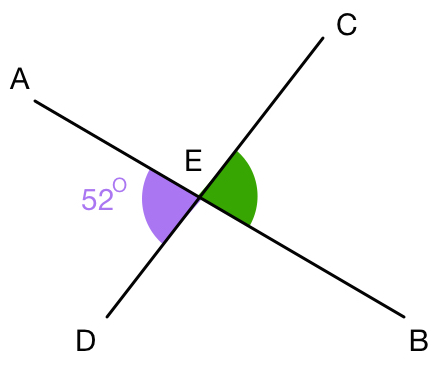In the above diagram, line segments $\overline{AB}$ and $\overline{CD}$ intersect at point $E.$ If $\angle AED={52}^\circ,$ what is the measure of $\angle CEB$ in degrees?

×